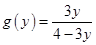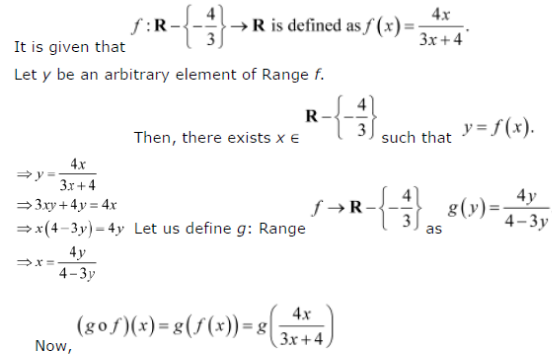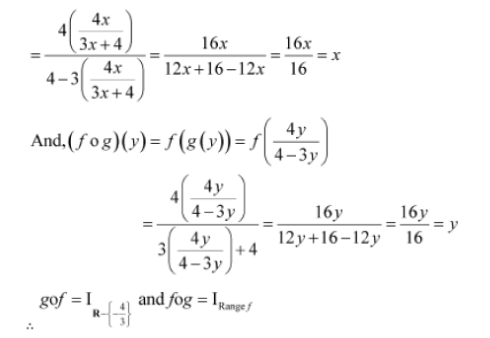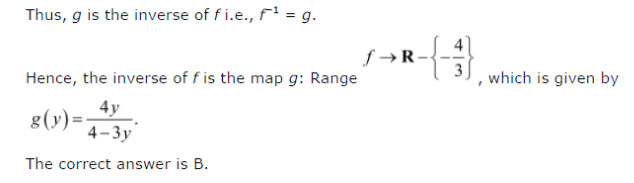# Let f : R - {-4/3} → R be a function defined as f(x) = 4x/3x + 4

Let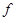: R –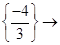R be a function defined as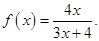The inverse ofis the map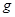: Range of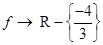given by:
(A)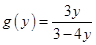(B)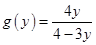( C)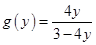(D)